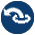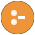#dicts.pl -- Dict utilities

This library defines utilities that operate on lists of dicts, notably to make lists of dicts consistent by adding missing keys, converting between lists of compounds and lists of dicts, joining and slicing lists of dicts.

dicts_same_tag(+List, -Tag) is semidet
True when List is a list of dicts that all have the tag Tag.
dict_keys(+Dict, -Keys) is det
True when Keys is an ordered set of the keys appearing in Dict.
dicts_same_keys(+List, -Keys) is semidet
True if List is a list of dicts that all have the same keys and Keys is an ordered set of these keys.
dicts_to_same_keys(+DictsIn, :OnEmpty, -DictsOut)
DictsOut is a copy of DictsIn, where each dict contains all keys appearing in all dicts of DictsIn. Values for keys that are added to a dict are produced by calling OnEmpty as below. The predicate dict_fill/4 provides an implementation that fills all new cells with a predefined value.
`call(:OnEmpty, +Key, +Dict, -Value)`
dict_fill(+ValueIn, +Key, +Dict, -Value) is det
Implementation for the dicts_to_same_keys/3 OnEmpty closure that fills new cells with a copy of ValueIn. Note that copy_term/2 does not really copy ground terms. Below are two examples. Note that when filling empty cells with a variable, each empty cell is bound to a new variable.
```?- dicts_to_same_keys([r{x:1}, r{y:2}], dict_fill(null), L).
L = [r{x:1, y:null}, r{x:null, y:2}].
?- dicts_to_same_keys([r{x:1}, r{y:2}], dict_fill(_), L).
L = [r{x:1, y:_G2005}, r{x:_G2036, y:2}].```

Use dict_no_fill/3 to raise an error if a dict is missing a key.

dicts_join(+Key, +DictsIn, -Dicts) is semidet
Join dicts in Dicts that have the same value for Key, provided they do not have conflicting values on other keys. For example:
```?- dicts_join(x, [r{x:1, y:2}, r{x:1, z:3}, r{x:2,y:4}], L).
L = [r{x:1, y:2, z:3}, r{x:2, y:4}].```
Errors
- `existence_error(key, Key, Dict)` if a dict in Dicts1 or Dicts2 does not contain Key.
dicts_join(+Key, +Dicts1, +Dicts2, -Dicts) is semidet
Join two lists of dicts (Dicts1 and Dicts2) on Key. Each pair D1-D2 from Dicts1 and Dicts2 that have the same (==) value for Key creates a new dict D with the union of the keys from D1 and D2, provided D1 and D2 to not have conflicting values for some key. For example:
```?- DL1 = [r{x:1,y:1},r{x:2,y:4}],
DL2 = [r{x:1,z:2},r{x:3,z:4}],
dicts_join(x, DL1, DL2, DL).
DL = [r{x:1, y:1, z:2}, r{x:2, y:4}, r{x:3, z:4}].```
Errors
- `existence_error(key, Key, Dict)` if a dict in Dicts1 or Dicts2 does not contain Key.
dicts_slice(+Keys, +DictsIn, -DictsOut) is det
DictsOut is a list of Dicts only containing values for Keys.
dicts_to_compounds(?Dicts, +Keys, :OnEmpty, ?Compounds) is semidet
True when Dicts and Compounds are lists of the same length and each element of Compounds is a compound term whose arguments represent the values associated with the corresponding keys in Keys. When converting from dict to row, OnEmpty is used to compute missing values. The functor for the compound is the same as the tag of the pair. When converting from dict to row and the dict has no tag, the functor `row` is used. For example:
```?- Dicts = [_{x:1}, _{x:2, y:3}],
dicts_to_compounds(Dicts, [x], dict_fill(null), Compounds).
Compounds = [row(1), row(2)].
?- Dicts = [_{x:1}, _{x:2, y:3}],
dicts_to_compounds(Dicts, [x,y], dict_fill(null), Compounds).
Compounds = [row(1, null), row(2, 3)].
?- Compounds = [point(1,1), point(2,4)],
dicts_to_compounds(Dicts, [x,y], dict_fill(null), Compounds).
Dicts = [point{x:1, y:1}, point{x:2, y:4}].```

When converting from Dicts to Compounds Keys may be computed by dicts_same_keys/2.

## Undocumented predicates

The following predicates are exported, but not or incorrectly documented.

dict_no_fill(Arg1, Arg2, Arg3)